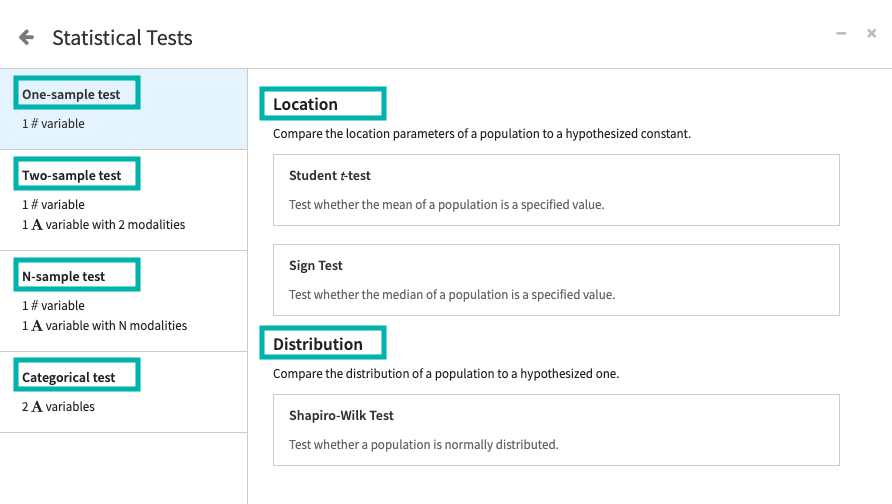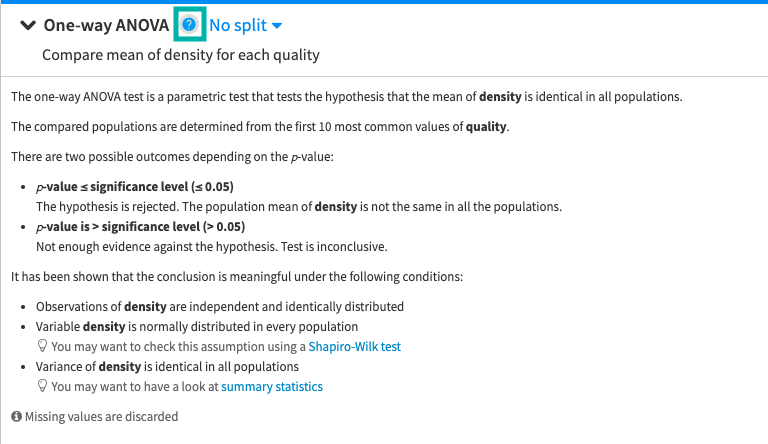# Concept: Test Categories¶

Dataiku DSS groups hypothesis tests into categories based on different test attributes.## 1. One-sample, Two-sample, and N-sample Tests¶

• One-sample tests consider one population from which a random sample is used to make inferences.

• Two-sample tests consider two populations from which independent random samples are used to make inferences.

• N-sample tests consider more than two populations with independent random samples that are used to make inferences.

## 2. Location or Distribution Tests¶

• Location tests evaluate hypotheses about location parameters. For example, the mean of a population (in the case of the one-sample Student’s t-test), and the median of a population (in the case of the Sign test).

• Distribution tests evaluate hypotheses about population distributions. For example, one-sample distribution tests compare the distribution of a population to a hypothesized one, and two-sample distribution tests compare the distributions of two populations.

## 3. Categorical Tests¶

DSS provides the Chi-square Independence test, to evaluate whether two categorical variables are independent.